# 41

## Summary

### Factorization

The number 41 is a prime number.

### Properties and families

Property or family Parameter values First few numbers Proof of satisfaction/membership/containment
prime number it is the 13th prime number 2, 3, 5, 7, 11, 13, 17, 19, 23, 29, 31, 37, 41, 43, 47, 53, 59, 61, 67, 71, 73, 79, 83, 89, 97, [SHOW MORE]View list on OEIS A natural numberis prime if and only ifis not divisible by any prime less than or equal to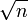. Sinceis between 6 and 7, we only need to check divisibility by primes less than or equal to 6, i.e., we need to verify that 41 is not divisible by the primes 2, 3, and 5.
regular prime first regular prime occurring after an irregular prime 3, 5, 7, 11, 13, 17, 19, 23, 29, 31, 41, 43, 47, 53, 61, [SHOW MORE]View list on OEIS
lucky number of Euler biggest of six such numbers 2, 3, 5, 11, 17, 41 View list on OEIS A primeis a lucky number of Euler iff the ring of integers in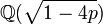is a unique factorization domain.
Proth prime: prime of the formwith3, 5, 13, 17, 41, 97, 113, [SHOW MORE]View list on OEIS

## Waring representations

### Sums of squares

Item Value
unique (up to plus/minus and ordering) representation as sum of two squares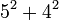. Note that existence and uniqueness both follow from it being a prime that is 1 mod 4.
This also corresponds to the factorizationin the ring of Gaussian integers.
representations as sum of three squares (up to ordering and plus/minus equivalence)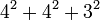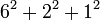## Prime-generating polynomials

Below are some polynomials that give prime numbers for small input values, which give the value 41 for suitable input choice.

Polynomial Degree Some values for which it generates primes Input valueat which it generates 412 all numbers 1-10, because 11 is one of the lucky numbers of Euler. 62 all numbers 1-40, because 41 is one of the lucky numbers of Euler. 1